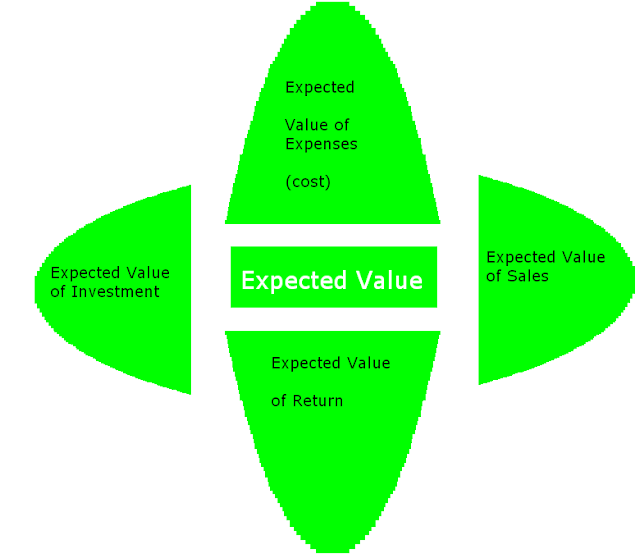222

## Latest \$type=blogging\$count=5\$author=hide\$comment=hide\$label=hide\$date=hide\$show=home

Expected value is the estimated value of any asset which is calculated on the basis of probability and other mathematical principles. All those who have good hand on maths can become good accounting expert. In accounting and finance, we need to estimate the investment. We invest our money on any project whose cost will be less. Without investing real amount, we can calculate its expected value and take better investment decisions.

Moreover with this, we can calculate the expected value of return and expected value of salable products.

Following are the simple steps to calculate Expected Value

1st Step : Identify Your Problem

First of all, you need to think about accounting problem. Which accounting or finance item's expected value, you need to calculate? For example, you need to calculate expected cost value, expected sale value or expected earning value.

2nd Step : Make the List of All Possible Results

For example, your problem is to calculate expected value of your earning. For example, you are expecting 50% (chance), you will gain 10% Profit. You are expecting 30% ( Chance), you will gain 20% profit. You are expecting 20% (Chance), you will gain 30% profit.

3rd Step : Calculate the Expected Value on the Basis of Probability Formula

Formula of  Expected Rate of Return =

(Probability of Outcome x Rate of Outcome) + (Probability of Outcome x Rate of Outcome)

= (.50 x .10) + (.30 x .20) + (.20 x .30)

=  0.05 + 0.06 + 0.06

0.17

For example, if investment is \$ 10,000, Now,

expected value of earning

=  \$ 10,000 X 0.17 = \$1700

On this expected value, businessman can make his plan of using same value.

### Related ResourcesFinance Latest Content

Which is Better Fixed Deposit or MIS?

Name

ltr
item
Accounting Education: Expected Value
Expected Value
http://4.bp.blogspot.com/--T8el6q6YiM/VbmypzlVzoI/AAAAAAAASNs/1snYhmSjjyA/s640/expected%2Bvalue.PNG
http://4.bp.blogspot.com/--T8el6q6YiM/VbmypzlVzoI/AAAAAAAASNs/1snYhmSjjyA/s72-c/expected%2Bvalue.PNG
Accounting Education
https://www.svtuition.org/2015/07/expected-value.html
https://www.svtuition.org/
https://www.svtuition.org/
https://www.svtuition.org/2015/07/expected-value.html
true
2410664366776677676
UTF-8